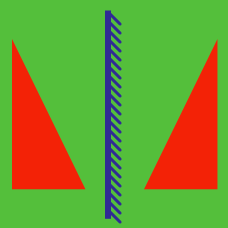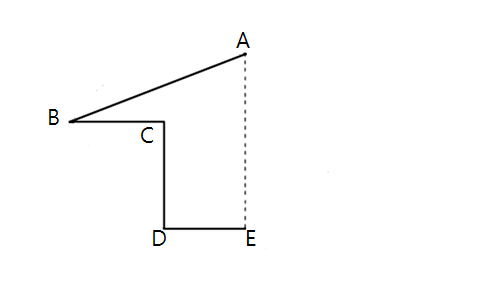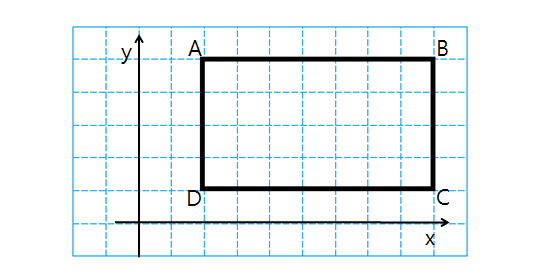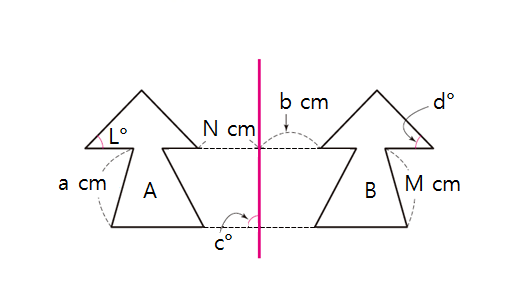Geometry

# ReflectionThe lengths of the lines in the above figure (a half house) are as follows: $\lvert \overline{AB} \rvert = 9, \lvert \overline{BC} \rvert = 3,$$\lvert \overline{CD} \rvert = 3, \lvert \overline{DE} \rvert = 3.$ If the shape is flipped across the line segment $$\overline{AE}$$ to complete a house, what will be the perimeter of the house?

The reflection of the circle $$x^2+y^2-20x-20y+196=0$$ in the origin $$(0,0)\$$ is $$\ x^2+y^2+ax+by+c=0.$$ What is $$a+b+c$$?

The point $$A=(-4,5)$$ is reflected in the line $$y=x.$$ What are the coordinates of the resulting image?If rectangle $$ABCD$$ in the above graph is reflected in the line $$y = -1,$$ what is the image of point $$D?$$ The side length of each square in the graph paper is $$1.$$In the above diagram, shape $$B$$ on the right is the reflection of shape $$A$$ in the red line. Given the following numbers: $L = 48, \quad N = 3, \quad M = 6$ in the diagram, what is the value of $$a - b + c - d?$$

×

Problem Loading...

Note Loading...

Set Loading...